Examples

Chapter 1 Class 12 Relation and Functions
Serial order wise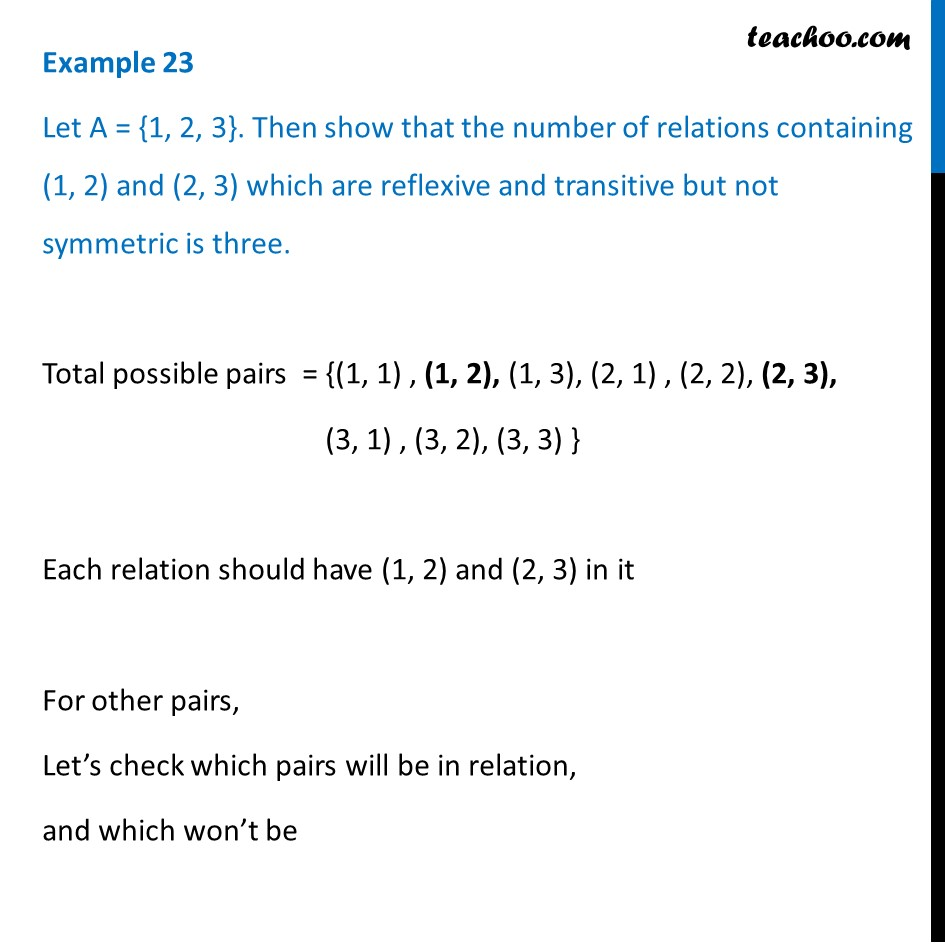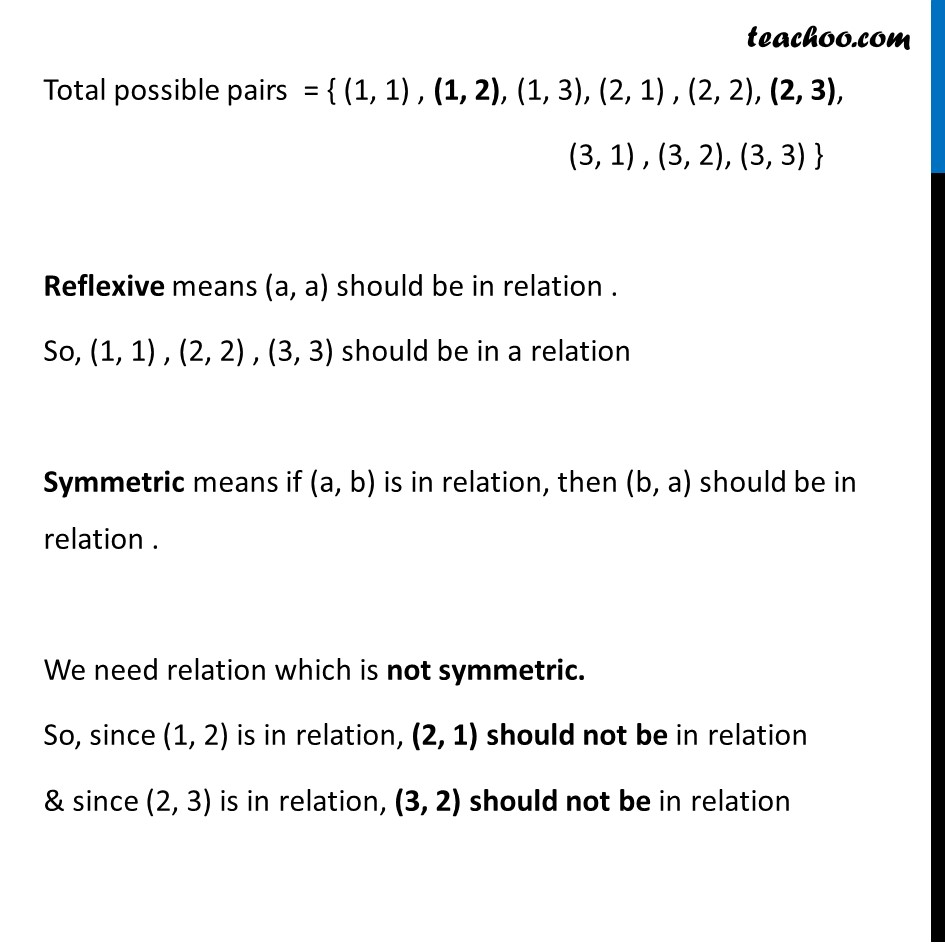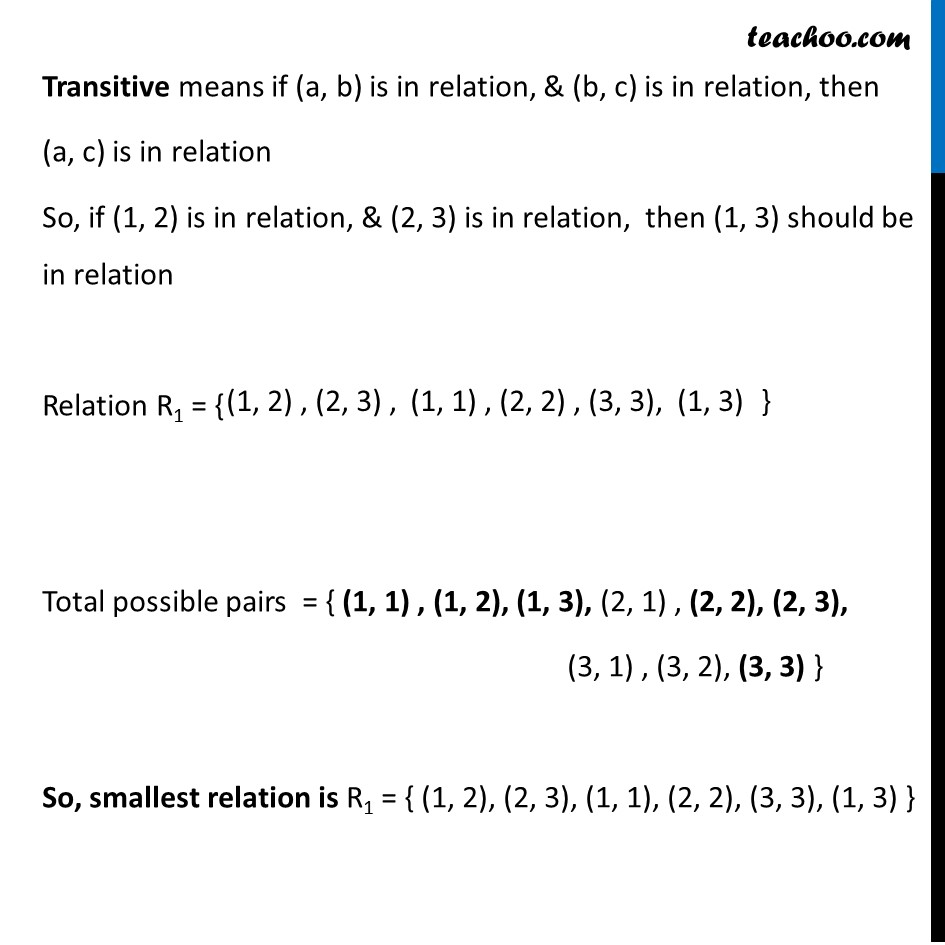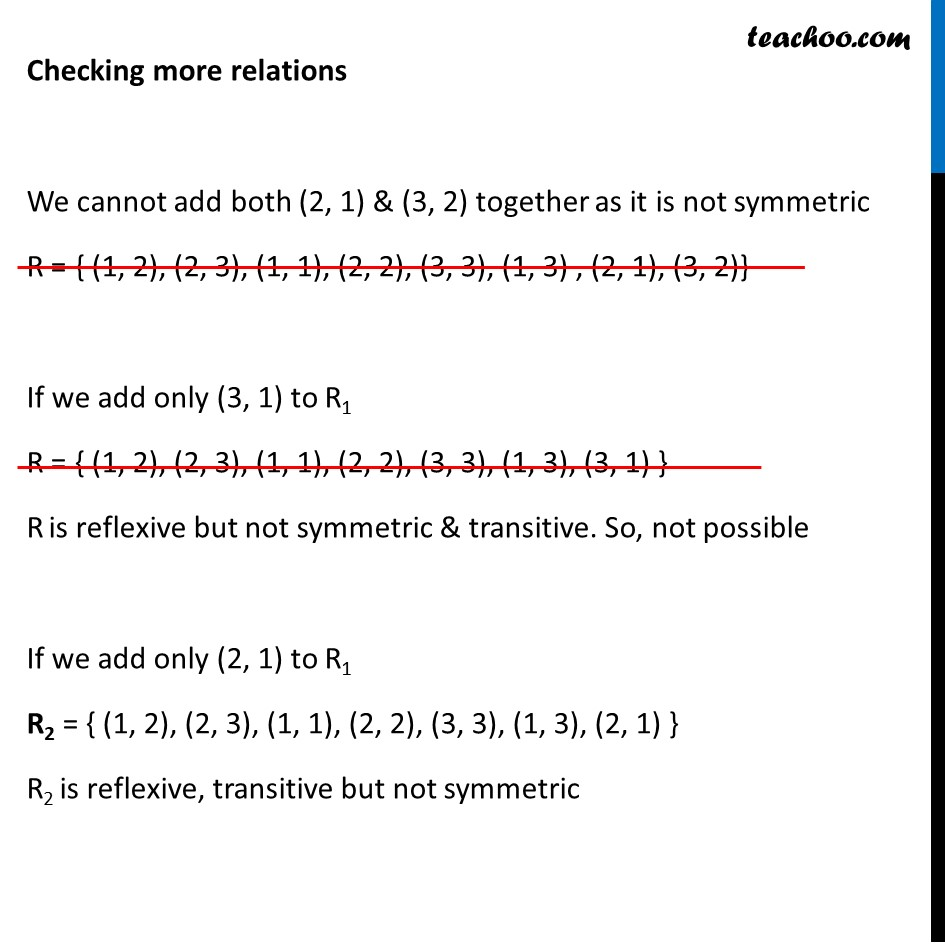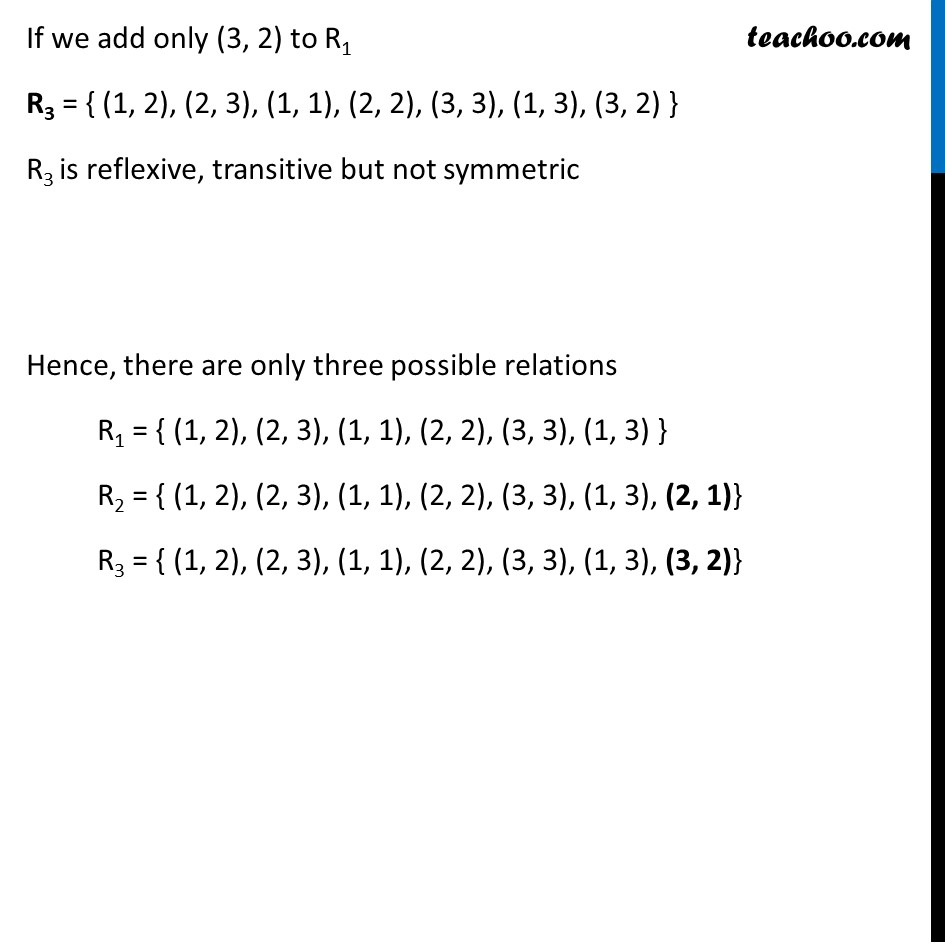Learn in your speed, with individual attention - Teachoo Maths 1-on-1 Class

### Transcript

Example 23 Let A = {1, 2, 3}. Then show that the number of relations containing (1, 2) and (2, 3) which are reflexive and transitive but not symmetric is three. Total possible pairs = {(1, 1) , (1, 2), (1, 3), (2, 1) , (2, 2), (2, 3), (3, 1) , (3, 2), (3, 3) } Each relation should have (1, 2) and (2, 3) in it For other pairs, Let’s check which pairs will be in relation, and which won’t be Total possible pairs = { (1, 1) , (1, 2), (1, 3), (2, 1) , (2, 2), (2, 3), (3, 1) , (3, 2), (3, 3) } Reflexive means (a, a) should be in relation . So, (1, 1) , (2, 2) , (3, 3) should be in a relation Symmetric means if (a, b) is in relation, then (b, a) should be in relation . We need relation which is not symmetric. So, since (1, 2) is in relation, (2, 1) should not be in relation & since (2, 3) is in relation, (3, 2) should not be in relation Transitive means if (a, b) is in relation, & (b, c) is in relation, then (a, c) is in relation So, if (1, 2) is in relation, & (2, 3) is in relation, then (1, 3) should be in relation Relation R1 = { Total possible pairs = { (1, 1) , (1, 2), (1, 3), (2, 1) , (2, 2), (2, 3), (3, 1) , (3, 2), (3, 3) } So, smallest relation is R1 = { (1, 2), (2, 3), (1, 1), (2, 2), (3, 3), (1, 3) } Checking more relations We cannot add both (2, 1) & (3, 2) together as it is not symmetric R = { (1, 2), (2, 3), (1, 1), (2, 2), (3, 3), (1, 3) , (2, 1), (3, 2)} If we add only (3, 1) to R1 R = { (1, 2), (2, 3), (1, 1), (2, 2), (3, 3), (1, 3), (3, 1) } R is reflexive but not symmetric & transitive. So, not possible If we add only (2, 1) to R1 R2 = { (1, 2), (2, 3), (1, 1), (2, 2), (3, 3), (1, 3), (2, 1) } R2 is reflexive, transitive but not symmetric If we add only (3, 2) to R1 R3 = { (1, 2), (2, 3), (1, 1), (2, 2), (3, 3), (1, 3), (3, 2) } R3 is reflexive, transitive but not symmetric Hence, there are only three possible relations R1 = { (1, 2), (2, 3), (1, 1), (2, 2), (3, 3), (1, 3) } R2 = { (1, 2), (2, 3), (1, 1), (2, 2), (3, 3), (1, 3), (2, 1)} R3 = { (1, 2), (2, 3), (1, 1), (2, 2), (3, 3), (1, 3), (3, 2)}